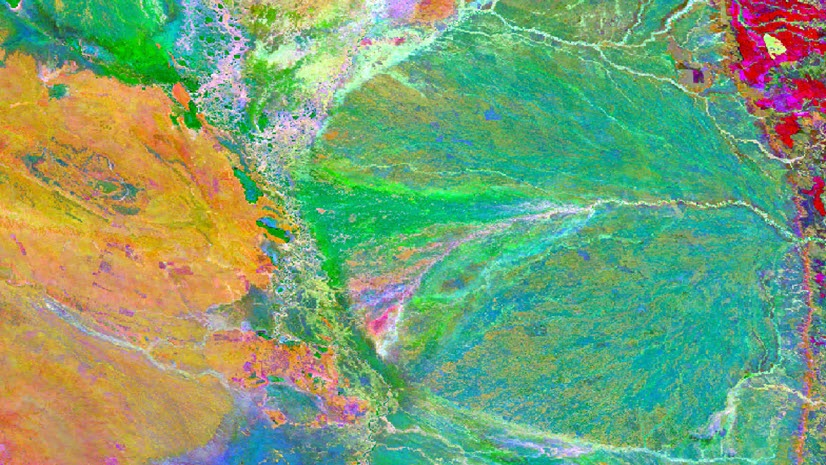Mapping

# Using rotation angles for markers, lines, or polygon fills

By Aileen Buckley, Mapping Center LeadIf having the proper orientation, or rotation angle is an important characteristic of your symbols, it is important to make sure your symbols accurately display the phenomenon they depict. Thus, I thought it would be a good idea to review the use of rotation angles in ArcMap.

You have probably set rotation angles for symbols, (markers, hash lines, and Line or gradient fills), your data frame, and maybe even for point features which can be rotated by the angle stored in a field. Most of you already know your own data well, but if you’ve
ever downloaded data or inherited legacy data, you may not know which way the rotation angles were calculated (so if you publish data, remember to invest a few minutes in authoring the metadata).

ArcGIS has tools and functionality that may use any of three rotation methods:  Geographic, Arithmetic, and Graphic.Usually in ArcMap there is a preview to show you how the rotation angle you have chosen will look, so it isn’t necessary to know which method is being used to complete most tasks. But, when you need to create data containing rotation angles for displaying symbols, you will need to be aware of which method is appropriate. For instance, rotating point feature symbology supports Geographic and Arithmetic rotation, so either will work. Cartographic representations, however, only support Graphic Rotation, and will assume your data’s rotation angles use that method. This is something you may need to address if you are overriding the rotation angle of a representation marker using a field value.

If you know which method was used to store your data attribute values’ rotation angle, you can convert from any method to any of the others using these calculations, which can be used as Advanced Field Calculator expressions:

Arithmetic to Graphic
a = [Angle]
d = 0
If a >= 0 And a <= 90 Then
d = 270 + a
ElseIf a > 90 And a <= 360 Then
d = a – 90
End If Geographic to Graphic
a = [Angle]
d = 0
d = 360 – a
Arithmetic to Geographic & vice-versa
a = [Angle]
d = 0
If a >= 0 And a <= 90 Then
d = 90 – a
ElseIf a > 90 And a <= 360 Then
d = 450 – a
End If monographic